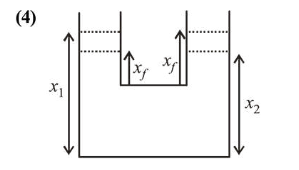# Two identical cylindrical vessels are kept on the ground and each contain the same liquid of density d.Question:

Two identical cylindrical vessels are kept on the ground and each contain the same liquid of density $d$. The area of the base of both vessels is $S$ but the height of liquid in one vessel is $x_{1}$ and in the other, $x_{2}$. When both cylinders are connected through a pipe of negligible volume very close to the bottom, the liquid flows from one vessel to the other until it comes to equilibrium at a new height. The change in energy of the system in the process is :

1. (1) $g d S\left(x_{2}^{2}+x_{1}^{2}\right)$

2. (2) $g d S\left(x_{2}+x_{1}\right)^{2}$

3. (3) $\frac{3}{4} g d S\left(x_{2}-x_{1}\right)^{2}$

4. (4) $\frac{1}{4} g d S\left(x_{2}-x_{1}\right)^{2}$

Correct Option: , 4

Solution:Initial potential energy,

$U_{1}=\left(\rho S x_{1}\right) g \cdot \frac{x_{1}}{2}+\left(\rho S x_{2}\right) g \cdot \frac{x_{2}}{2}$

Final potential energy,

$U_{f}=\left(\rho S x_{f}\right) g \cdot \frac{x_{f}}{2} \times 2$

By volume conservation,

$S x_{1}+S x_{2}=S\left(2 x_{f}\right)$

$x_{f}=\frac{x_{1}+x_{2}}{2}$

When valve is opened loss in potentail energy occur till water level become same.

$\Delta U=U_{i}-U_{f}$

$\Delta U=\rho S g\left[\left(\frac{x_{1}^{2}}{2}+\frac{x_{2}^{2}}{2}\right)-x_{f}^{2}\right]$

$=\rho S g\left[\frac{x_{1}^{2}}{2}+\frac{x_{2}^{2}}{2}-\left(\frac{x_{1}+x_{2}}{2}\right)^{2}\right]$

$=\frac{\rho S g}{2}\left[\frac{x_{1}^{2}}{2}+\frac{x_{2}^{2}}{2}-x_{1} x_{2}\right]=\frac{\rho S g}{4}\left(x_{1}-x_{2}\right)^{2}$